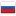Editorial of Educational Codeforces Round 16

Revision en6, by Edvard, 2016-08-30 01:56:47

### 710A - King Moves

Easy to see that there are only three cases in this problem. If the king is in the corner of the board the answer is 3. If the king is on the border of the board but not in a corner then the answer is 5. Otherwise the answer is 8.

С++ solution

Complexity: O(1).

### 710B - Optimal Point on a Line

The function of the total distance is monotonic between any pair of adjacent points from the input, so the answer is always in some of the given points. We can use that observation to solve the problem by calculating the total distance for each point from the input and finding the optimal point.

The other solution uses the observation that the answer is always is the middle point (by index) in the sorted list of the given points. The last fact is also can be easily proven.

C++ solution

Complexity: O(nlogn).

### 710C - Magic Odd Square

The problem was suggested by Resul Hangeldiyev Resul.

The solution can be got from the second sample testcase. Easy to see that if we place all odd numbers in the center in form of rhombus we will get a magic square.

C++ solution

Complexity: O(n2).

### 710D - Two Arithmetic Progressions

I wanted to give this problem a lot of time ago. I thought it is very standard problem, but I underestimated its difficulty.

Let's write down the equation describing the problem: a1k + b1 = a2l + b2 → a1k - a2l = b2 - b1. So we have linear Diofant equation with two variables: Ax + By = C, A = a1, B =  - a2, C = b2 - b1. The solution has the form:, where the last equation can be solved by extended Euclid algorithm and p is any integral number. The variable p should satisfy two conditions:and. The values A and B are fixed, so we can get the segment of possible values for the values p. The length of the segment is the answer for the problem.

C++ solution

Complexity: O(log(max(a1, a2))).

### 710E - Generate a String

The problem was suggested by Zi Song Yeoh zscoder.

This problem has a simple solution described by participants in the comments.

My solution is a little harder. Let's solve it using dynamic programming. Let zn be the smallest amount of time needed to get n letters 'a'. Let's consider transitions: the transition for adding one letter 'a' can be simply done. Let's process transitions for multiplying by two and subtraction by one simultaneously: let's decrease the number i i times by one right after getting it. Easy to see that such updates never include each other, so we can store them in queue by adding the new update at the tail of the queue and taking the best update from the head.

The solution is hard to describe, but it is very simple in the code, so please check it to understand the idea :-)

C++ solution

Complexity: O(n).

### 710F - String Set Queries

The problem was suggested by Alexandr Kulkov adamant.

Let's get rid of the queries for deleting a string. There are no strings that will be added two times, so we can calculate the answer for the added (but not deleted strings) and for the deleted separately and subtract the second from the first to get the answer. So we can consider that there are no queries of deletion.

Now let's use Aho-Corasik algorithm. The only difficulty is that the strings are adding in online mode, but Aho-Corasik algorithm works only after adding all the strings. Note that the answer for the given set of strings equal to the answer for any part of the set plus the answer for the remaining part. Let's use the trick with converting the static data structure (Aho-Corasik in this case) to the dynamic one.

For the set of n strings let's maintain a set of no more than logn sets of the strings with sizes of different powers of two. After adding new string we should move the sets from the lowest powers of two to the largest until we got an invariant set of sets.

Easy to see that each string will be moved no more than logm times, so we can process each query in O(logn) time.

C++ solution

Complexity: O((slen + m)logm), where slen is the total length of the string from the input.#### History

RevisionsRev. Lang. By When Δ Comment
en6Edvard 2016-08-30 01:56:47 4835
en5Edvard 2016-08-30 01:11:02 1545
en4Edvard 2016-08-30 00:41:38 1965
en3Edvard 2016-08-30 00:04:38 2 Tiny change: 'ncorner than the answ' -> 'ncorner then the answ'
en2Edvard 2016-08-30 00:03:23 945
en1Edvard 2016-08-29 23:57:58 1839 Initial revision for English translation
ru4Edvard 2016-08-25 01:51:17 4900 Мелкая правка: 'ность: $O(slen\cdot logm)$, гд' -
ru3Edvard 2016-08-25 01:37:55 1633
ru2Edvard 2016-08-25 01:24:39 1967 Мелкая правка: 'уравнение получается решается ' -> 'уравнение решается '
ru1Edvard 2016-08-25 01:08:13 2829 Первая редакция (опубликовано)